Updating search results...

# 12 Results

View
Selected filters:
• NC.M1.A-SSE.1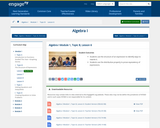Conditional Remix & Share Permitted
CC BY-NC-SA
Rating
0.0 stars

Students use the structure of an expression to identify ways to rewrite it. Students use the distributive property to prove equivalency of expressions.

Subject:
Math 1
Mathematics
Material Type:
Lesson
Author:
Engage NY
01/31/2020Conditional Remix & Share Permitted
CC BY-NC-SA
Rating
0.0 stars

CK-12 Foundation's Algebra FlexBook is an introduction to algebraic concepts for the high school student. Topics include: Equations & Functions, Real Numbers, Equations of Lines, Solving Systems of Equations & Quadratic Equations.

Subject:
Mathematics
Material Type:
Textbook
Provider:
CK-12 Foundation
Provider Set:
CK-12 FlexBook
12/06/2018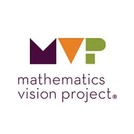Unrestricted Use
CC BY
Rating
0.0 stars

The focus of this task is on the generation of multiple expressions that connect with the visuals provided for the checkerboard borders. These expressions will also provide opportunity to discuss equivalent expressions and review the skills students have previously learned about simplifying expressions and using variables.

Subject:
Math 1
Mathematics
Material Type:
Activity/Lab
Author:
The Mathematics Assessment Project
02/23/2020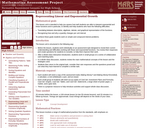Only Sharing Permitted
CC BY-NC-ND
Rating
0.0 stars

This lesson unit is intended to help teachers assess how well students are able to interpret exponential and linear functions and in particular to identify and help students who have the following difficulties: translating between descriptive, algebraic and tabular data, and graphical representation of the functions; recognizing how, and why, a quantity changes per unit intervale; and to achieve these goals students work on simple and compound interest problems.

Subject:
Algebra
Geometry
Mathematics
Material Type:
Assessment
Lesson Plan
Provider:
Shell Center for Mathematical Education
Provider Set:
Mathematics Assessment Project (MAP)
06/24/2019Unrestricted Use
CC BY
Rating
0.0 stars

In the context of predicting the account balance at different times for an account earning
5% interest annually, students examine the role of positive and negative integer exponents as well
as the need for rational exponents. Tables, graphs and reasoning based on the definition of radicals
and rules of exponents are used to attach meaning to using fractions such as ½ or ¾ as exponents.

Subject:
Math 1
Mathematics
Material Type:
Activity/Lab
Author:
The Mathematics Vision Project
02/23/2020Unrestricted Use
CC BY
Rating
0.0 stars

This task surfaces the idea that data exists on the intervals between the whole number
increments of a continuously increasing exponential function. Students will consider potential
strategies for calculating this data at equal fractional increments so that the multiplicative pattern
inherent in exponential functions is maintained.

Subject:
Math 1
Mathematics
Material Type:
Activity/Lab
Author:
The Mathematics Vision Project
02/23/2020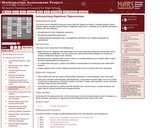Only Sharing Permitted
CC BY-NC-ND
Rating
0.0 stars

This lesson unit is intended to help teachers assess how well students are able to translate between words, symbols, tables, and area representations of algebraic expressions. It will help teachers to identify and support students who have difficulty in: recognizing the order of algebraic operations; recognizing equivalent expressions; and understanding the distributive laws of multiplication and division over addition (expansion of parentheses).

Subject:
Algebra
Mathematics
Material Type:
Assessment
Lesson Plan
Provider:
Shell Center for Mathematical Education
Provider Set:
Mathematics Assessment Project (MAP)
06/24/2019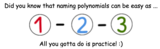Unrestricted Use
CC BY
Rating
0.0 stars

This a remix from original author Nancy Hetrick. The original resource did not have a practive video&nbsp;that worked so I made a 5 question Quizlet to review.As students prepare to work with algebraic expressions, either with operations (add, subtract, multiply, or divide) or when making connections to constant, linear, and/or quadratic functions, it is important that students can name basic polynomial expressions as monomials, binomials, or trinomials, During this activity students will learn how to name these polynomials through watching a video and then&nbsp;practice naming polynomials through a Quizlet.

Subject:
Algebra
Math 1
Material Type:
Activity/Lab
Author:
KORI ELLIOTT
07/08/2020Rating
0.0 stars

Students will continue to interpret expressions, create equations, rewrite equations and functions in different but equivalent forms, and graph and interpret functions, but this time using polynomial functions, and more specifically quadratic functions, as well as square root and cube root functions.

Subject:
Math 1
Math 2
Math 3
Mathematics
Material Type:
Unit of Study
Provider:
EngageNY
Author:
EngageNY
02/26/2019Unrestricted Use
CC BY
Rating
0.0 stars

This task provides opportunities for students to become fluent converting between
exponential and radical representations of expressions, as well as using the rules of exponents to

Subject:
Math 1
Mathematics
Material Type:
Activity/Lab
Author:
The Mathematics Vision Project
02/23/2020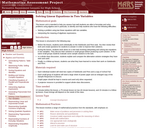Only Sharing Permitted
CC BY-NC-ND
Rating
0.0 stars

This lesson unit is intended to help teachers assess how well students are able to formulate and solve problems using algebra and, in particular, to identify and help students who have the following difficulties: solving a problem using two linear equations with two variables; and interpreting the meaning of algebraic expressions.

Subject:
Algebra
Mathematics
Material Type:
Assessment
Lesson Plan
Provider:
Shell Center for Mathematical Education
Provider Set:
Mathematics Assessment Project (MAP)
07/31/2019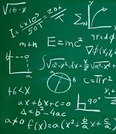Unrestricted Use
CC BY
Rating
0.0 stars

Items that I have used when teaching this unit.&nbsp;

Subject:
Math 1
Material Type:
Unit of Study
Author:
Wendi Hinson